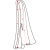## Viscose Stole

• 1.
Stylish & Designer stole
• 2.
Pocket Friendly Price
• 3.
Assured Quality Satisfaction
Showing 1 to 42 of 45 (2 Pages)
Show:
Sort By:
Rs. 540.00
• Size
• One Size
Rs. 845.00
• Size
• One Size
Rs. 540.00
• Size
• One Size
Rs. 1,080.00
• Size
• One Size
Rs. 810.00
• Size
• One Size
Rs. 810.00
• Size
• One Size
Rs. 1,080.00
• Size
• One Size
Rs. 900.00
• Size
• One Size
Rs. 2,700.00
• Size
• One Size
Rs. 1,800.00
• Size
• One Size
Rs. 900.00
• Size
• One Size
Rs. 810.00
• Size
• One Size
Rs. 720.00
• Size
• One Size
Rs. 720.00
• Size
• One Size
Rs. 540.00
• Size
• One Size
Rs. 1,495.00
• Size
• One Size
Women Stole Heavy Embroidery
Satisfaction Assured
SKU: EM2126
Rs. 875.00
• Size
• One Size
Rs. 425.00
• Size
Rs. 995.00
• Size
• One Size
Rs. 995.00
• Size
• One Size
Rs. 995.00
• Size
• One Size
Rs. 395.00
• Size
• One Size
Rs. 850.00
• Size
• One Size
Rs. 495.00
• Size
• One Size
Rs. 1,440.00
• Size
• One Size
Rs. 785.00
• Size
• One Size
Rs. 595.00
• Size
• One Size
Rs. 695.00
• Size
• One Size
Rs. 795.00
• Size
• One Size
Rs. 750.00
• Size
• One Size
Rs. 1,395.00
• Size
• One Size
Rs. 795.00
• Size
• One Size
Rs. 795.00
• Size
• One Size
Rs. 645.00
• Size
• One Size
Rs. 595.00
• Size
• One Size
Rs. 675.00
• Size
• One Size
Rs. 695.00
• Size
• One Size
Rs. 695.00
• Size
• One Size
Rs. 686.00
• Size
• One Size
Rs. 800.00
• Size
• One Size
Rs. 1,750.00
• Size
• One Size
Women self desigan printed border
Satisfaction Assured
SKU: em2820
Rs. 1,395.00